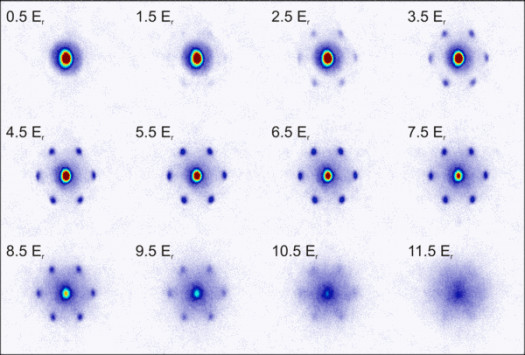# What Do You Know About Positive Exponents?

Approved & Edited by ProProfs Editorial Team
At ProProfs Quizzes, our dedicated in-house team of experts takes pride in their work. With a sharp eye for detail, they meticulously review each quiz. This ensures that every quiz, taken by over 100 million users, meets our standards of accuracy, clarity, and engagement.
| Written by Livyn
L
Livyn
Community Contributor
Quizzes Created: 348 | Total Attempts: 140,973
Questions: 10 | Attempts: 131SettingsAn exponent is a number having a power. If the power of an exponent is positive then it is called as positive exponent. Positive Exponents is nothing but the power of a number is to be positive.

• 1.

### There are how many kinds of exponents?

• A.

One

• B.

Two

• C.

Three

• D.

Five

B. Two
Explanation
The question asks how many kinds of exponents there are. The answer is two because there are two main types of exponents: positive and negative. Positive exponents indicate multiplication, while negative exponents indicate division. So, there are two kinds of exponents based on their mathematical operations.

Rate this question:

• 2.

### Positive Exponents is sub-topic under ...

• A.

Decimals

• B.

Equations

• C.

Exponents

• D.

Ratio

C. Exponents
Explanation
The sub-topic "Positive Exponents" falls under the broader topic of "Exponents". Exponents involve raising a number to a certain power, and positive exponents specifically refer to when the power is a positive integer. This sub-topic would cover concepts such as multiplying numbers with positive exponents, dividing numbers with positive exponents, and simplifying expressions with positive exponents.

Rate this question:

• 3.

### Exponents is a topic under ....

• A.

Algebra

• B.

Geometry

• C.

Decimals

• D.

Square roots

A. Algebra
Explanation
Exponents is a topic under algebra because it involves the manipulation and simplification of powers and exponents. In algebra, exponents are used to represent repeated multiplication and to simplify expressions by combining like terms. Understanding exponents is crucial in solving equations, graphing functions, and working with polynomials.

Rate this question:

• 4.

### Algebra is a topic under ....

• A.

Mathematics

• B.

Music

• C.

Creative arts

• D.

Literature

A. Mathematics
Explanation
Algebra is a branch of mathematics that deals with symbols and the rules for manipulating those symbols. It involves solving equations, simplifying expressions, and understanding patterns and relationships between numbers. Therefore, it is clear that algebra is a topic under mathematics.

Rate this question:

• 5.

### Mathematics is usually taught in ....

• A.

School

• B.

Church

• C.

Mosque

• D.

Restaurant

A. School
Explanation
Mathematics is usually taught in school because it is a fundamental subject that provides a foundation for many other fields of study. Schools have dedicated math teachers and resources to effectively teach and assess students' mathematical skills and knowledge. Additionally, schools provide a structured learning environment where students can engage in math-related activities, such as problem-solving and group discussions, to enhance their understanding of mathematical concepts. Teaching mathematics in school ensures that students receive a comprehensive education and develop the necessary skills for future academic and professional pursuits.

Rate this question:

• 6.

### Exponents are also called

• A.

Powers

• B.

Ratios

• C.

Decimals

• D.

Roots

A. Powers
Explanation
Exponents are also called powers because they represent the number of times a base number is multiplied by itself. In other words, an exponent tells us how many times to multiply the base number. For example, in the expression 2^3, the base number is 2 and the exponent is 3. This means that we need to multiply 2 by itself three times: 2 x 2 x 2 = 8. So, powers and exponents are interchangeable terms used to describe this mathematical concept.

Rate this question:

• 7.

### Mathematics is studied by ....

• A.

Humans

• B.

Toys

• C.

Animals

• D.

Aliens

A. Humans
Explanation
Mathematics is a subject that is studied and understood by humans. It involves the use of logical reasoning, problem-solving skills, and mathematical concepts that are specifically designed for human cognition. While toys may be used to teach basic mathematical concepts to young children, animals and aliens do not possess the cognitive abilities to comprehend and study mathematics as humans do. Therefore, the correct answer is humans.

Rate this question:

• 8.

### A negative exponent means how many timesto .... one by the number.

• A.

Divide

• B.

• C.

Multiply

• D.

Subtract

C. Multiply
Explanation
A negative exponent means how many times to multiply one by the number.

Rate this question:

• 9.

### Who is not a mathemacian among them?

• A.

Pythagoras

• B.

Albert Einstein

• C.

Will Smith

• D.

Isaac Newton

C. Will Smith
Explanation
Will Smith is not a mathematician. Pythagoras, Albert Einstein, and Isaac Newton are all famous mathematicians who have made significant contributions to the field of mathematics. Will Smith, on the other hand, is a well-known actor and musician, not known for his work in mathematics.

Rate this question:

• 10.

### Mathematics is a course or subject taught in ....

• A.

Restaurant

• B.

Museum

• C.

Factory

• D.

SchoolBack to top